# Python分析和实现基于用户和Item的协同过滤算法打开微信扫一扫，关注《数据与算法联盟》

1：协同过滤算法简介

2：协同过滤算法的核心

3：协同过滤算法的应用方式

4：基于用户的协同过滤算法实现

5：基于物品的协同过滤算法实现

# 一：协同过滤算法简介

关于协同过滤的一个最经典的例子就是看电影，有时候不知道哪一部电影是我们喜欢的或者评分比较高的，那么通常的做法就是问问周围的朋友，看看最近有什么好的电影推荐。在问的时候，都习惯于问跟自己口味差不 多的朋友，这就是协同过滤的核心思想。

协同过滤是在海量数据中挖掘出小部分与你品味类似的用户，在协同过滤中，这些用户成为邻居，然后根据他们喜欢的东西组织成一个排序的目录推荐给你。所以就有如下两个核心问题

（1）如何确定一个用户是否与你有相似的品味？

（2）如何将邻居们的喜好组织成一个排序目录？

协同过滤算法的出现标志着推荐系统的产生，协同过滤算法包括基于用户和基于物品的协同过滤算法。

# 二：协同过滤算法的核心

要实现协同过滤，需要进行如下几个步骤

1）收集用户偏好

2）找到相似的用户或者物品

3）计算并推荐

# 三：协同过滤算法的应用方式

## 1：基于用户的协同过滤算法

### (1)：计算用户的相似度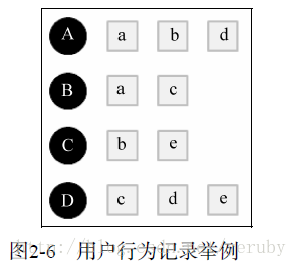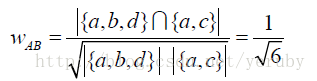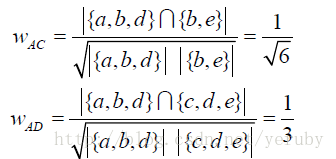但是这样计算的效率是低的，因为我们需要计算每一对用户之间的相似度，事实上，很多用户相互之间并没有对同样的物品产生过行为，所以很多时候当分子为0的时候没有必要再去计算分母，所以这里可以优化：即首先计算出|N(u) 并 N(v)| != 0 的用户对(u,v)，然后对这种情况计算分母以得到两个用户的相似度。

（1）建立物品到用户的倒查表T，表示该物品被哪些用户产生过行为；

（2）根据倒查表T，建立用户相似度矩阵W：在T中，对于每一个物品i，设其对应的用户为j,k，在W中，更新相应的元素值，w[j][k]=w[j][k]+1，w[k][j]=w[k][j]+1，以此类推，扫描完倒查表T中的所有物品后，就可以得到最终的用户相似度矩阵W，这里的W是余弦相似度中的分子部分，然后将W除以分母可以得到最终的用户兴趣相似度。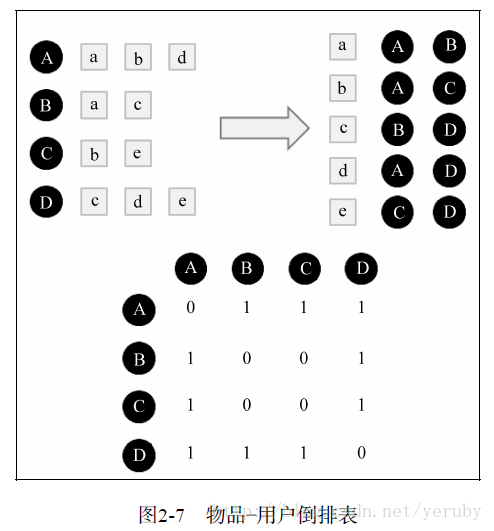### (2)：给用户推荐兴趣最相近的k个用户所喜欢的物品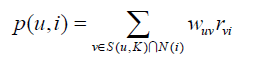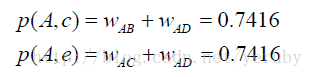## 2：基于物品的协同过滤算法(1)：构建物品的同现矩阵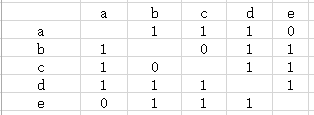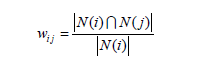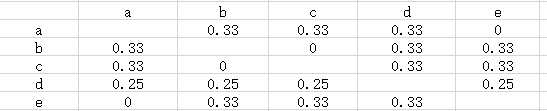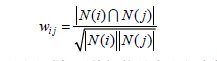(2)：建立用户对物品的评分矩阵（以A举例，没有评分的物品为0）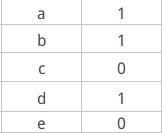(3)：矩阵计算推荐结果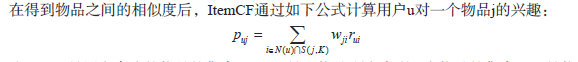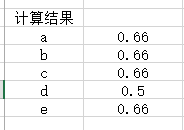## 3：混合推荐

1.用户i参与评分的项目集合为IiIi，用户j参与评分的项目集合为IjIj，找到它们的并集Uij=Ii∪IjUij=Ii∪Ij

2.在集合UijUij中用户i未评分的项目是Ni=Uij−IiNi=Uij−Ii，采用item-based CF方法重新估计用户i对NiNi中每个项目的评分。

3.这样用户i和j对UijUij的评分就都是非0值了，在此情况下计算他们的相似度。

# 四：基于用户的协同过滤算法实现

#-*-coding:utf-8-*-
'''
Created on 2016年5月2日

@author: Gamer Think
'''
from math import sqrt

fp = open("uid_score_bid","r")

users = {}

for line in open("uid_score_bid"):
lines = line.strip().split(",")
if lines not in users:
users[lines] = {}
users[lines][lines]=float(lines)

#----------------新增代码段END----------------------

class recommender:
#data：数据集，这里指users
#k：表示得出最相近的k的近邻
#metric：表示使用计算相似度的方法
#n：表示推荐book的个数
def __init__(self, data, k=3, metric='pearson', n=12):

self.k = k
self.n = n
self.username2id = {}
self.userid2name = {}
self.productid2name = {}

self.metric = metric
if self.metric == 'pearson':
self.fn = self.pearson
if type(data).__name__ == 'dict':
self.data = data

def convertProductID2name(self, id):

if id in self.productid2name:
return self.productid2name[id]
else:
return id

#定义的计算相似度的公式，用的是皮尔逊相关系数计算方法
def pearson(self, rating1, rating2):
sum_xy = 0
sum_x = 0
sum_y = 0
sum_x2 = 0
sum_y2 = 0
n = 0
for key in rating1:
if key in rating2:
n += 1
x = rating1[key]
y = rating2[key]
sum_xy += x * y
sum_x += x
sum_y += y
sum_x2 += pow(x, 2)
sum_y2 += pow(y, 2)
if n == 0:
return 0

#皮尔逊相关系数计算公式
denominator = sqrt(sum_x2 - pow(sum_x, 2) / n)  * sqrt(sum_y2 - pow(sum_y, 2) / n)
if denominator == 0:
return 0
else:
return (sum_xy - (sum_x * sum_y) / n) / denominator

def computeNearestNeighbor(self, username):
distances = []
for instance in self.data:
if instance != username:
distance = self.fn(self.data[username],self.data[instance])
distances.append((instance, distance))

distances.sort(key=lambda artistTuple: artistTuple,reverse=True)
return distances

#推荐算法的主体函数
def recommend(self, user):
#定义一个字典，用来存储推荐的书单和分数
recommendations = {}
#计算出user与所有其他用户的相似度，返回一个list
nearest = self.computeNearestNeighbor(user)
# print nearest

userRatings = self.data[user]
#         print userRatings
totalDistance = 0.0
#得住最近的k个近邻的总距离
for i in range(self.k):
totalDistance += nearest[i]
if totalDistance==0.0:
totalDistance=1.0

#将与user最相近的k个人中user没有看过的书推荐给user，并且这里又做了一个分数的计算排名
for i in range(self.k):

#第i个人的与user的相似度，转换到[0,1]之间
weight = nearest[i] / totalDistance

#第i个人的name
name = nearest[i]

#第i个用户看过的书和相应的打分
neighborRatings = self.data[name]

for artist in neighborRatings:
if not artist in userRatings:
if artist not in recommendations:
recommendations[artist] = (neighborRatings[artist] * weight)
else:
recommendations[artist] = (recommendations[artist]+ neighborRatings[artist] * weight)

recommendations = list(recommendations.items())
recommendations = [(self.convertProductID2name(k), v)for (k, v) in recommendations]

#做了一个排序
recommendations.sort(key=lambda artistTuple: artistTuple, reverse = True)

return recommendations[:self.n],nearest

def adjustrecommend(id):
bookid_list = []
r = recommender(users)
k,nearuser = r.recommend("%s" % id)
for i in range(len(k)):
bookid_list.append(k[i])
return bookid_list,nearuser[:15]        #bookid_list推荐书籍的id，nearuser[:15]最近邻的15个用户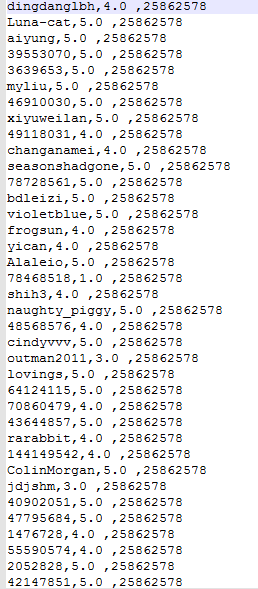bookid_list,near_list = adjustrecommend("changanamei")
print ("bookid_list:",bookid_list)
print ("near_list:",near_list)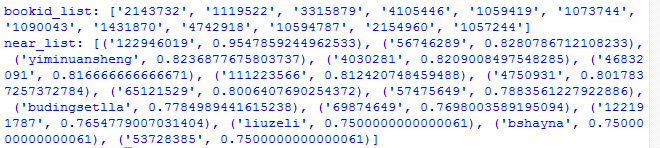# 五：基于物品的协同过滤算法的实现

#-*-coding:utf-8-*-

'''
Created on 2016-5-30

@author: thinkgamer
'''
import math

class ItemBasedCF:
def __init__(self,train_file):
self.train_file = train_file
self.readData()
def readData(self):
#读取文件，并生成用户-物品的评分表和测试集
self.train = dict()     #用户-物品的评分表
for line in open(self.train_file):
# user,item,score = line.strip().split(",")
user,score,item = line.strip().split(",")
self.train.setdefault(user,{})
self.train[user][item] = int(float(score))

def ItemSimilarity(self):
#建立物品-物品的共现矩阵
C = dict()  #物品-物品的共现矩阵
N = dict()  #物品被多少个不同用户购买
for user,items in self.train.items():
for i in items.keys():
N.setdefault(i,0)
N[i] += 1
C.setdefault(i,{})
for j in items.keys():
if i == j : continue
C[i].setdefault(j,0)
C[i][j] += 1
#计算相似度矩阵
self.W = dict()
for i,related_items in C.items():
self.W.setdefault(i,{})
for j,cij in related_items.items():
self.W[i][j] = cij / (math.sqrt(N[i] * N[j]))
return self.W

#给用户user推荐，前K个相关用户
def Recommend(self,user,K=3,N=10):
rank = dict()
action_item = self.train[user]     #用户user产生过行为的item和评分
for item,score in action_item.items():
for j,wj in sorted(self.W[item].items(),key=lambda x:x,reverse=True)[0:K]:
if j in action_item.keys():
continue
rank.setdefault(j,0)
rank[j] += score * wj
return dict(sorted(rank.items(),key=lambda x:x,reverse=True)[0:N])

#声明一个ItemBased推荐的对象
Item = ItemBasedCF("uid_score_bid")
Item.ItemSimilarity()
recommedDic = Item.Recommend("xiyuweilan")
for k,v in recommedDic.iteritems():
print k,"\t",v

1080309     8.18161438413
1119522     11.8165100292
1040104     7.92927319995
1254588     12.8331124639
1082138     7.72409411532
3131626     11.3426906217
26669243     6.96972128519
26305561     6.24816554216
26384985     6.36064881658
1085799     8.20314297616